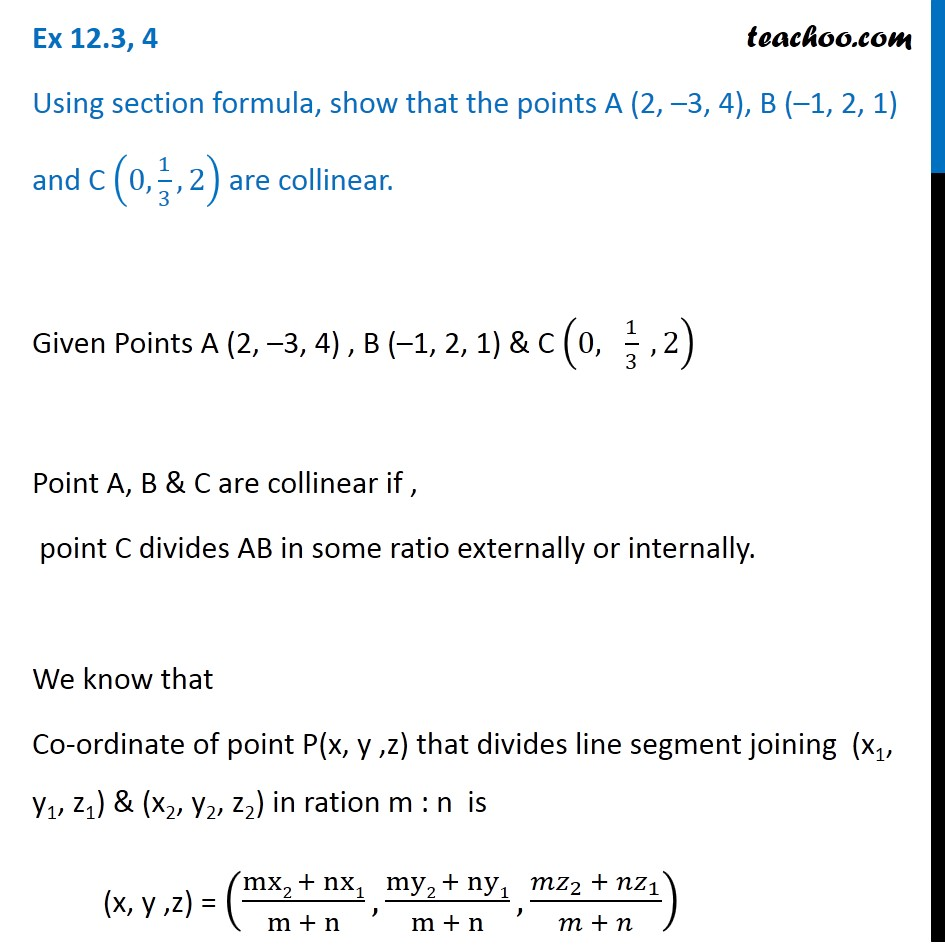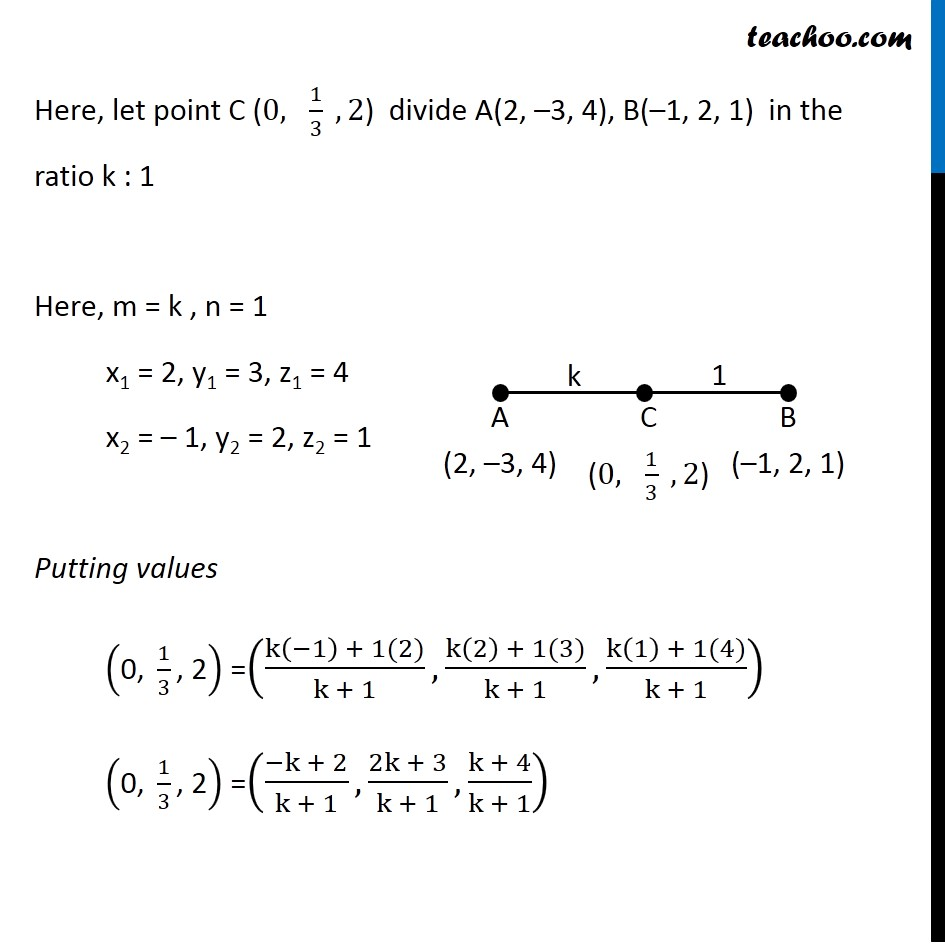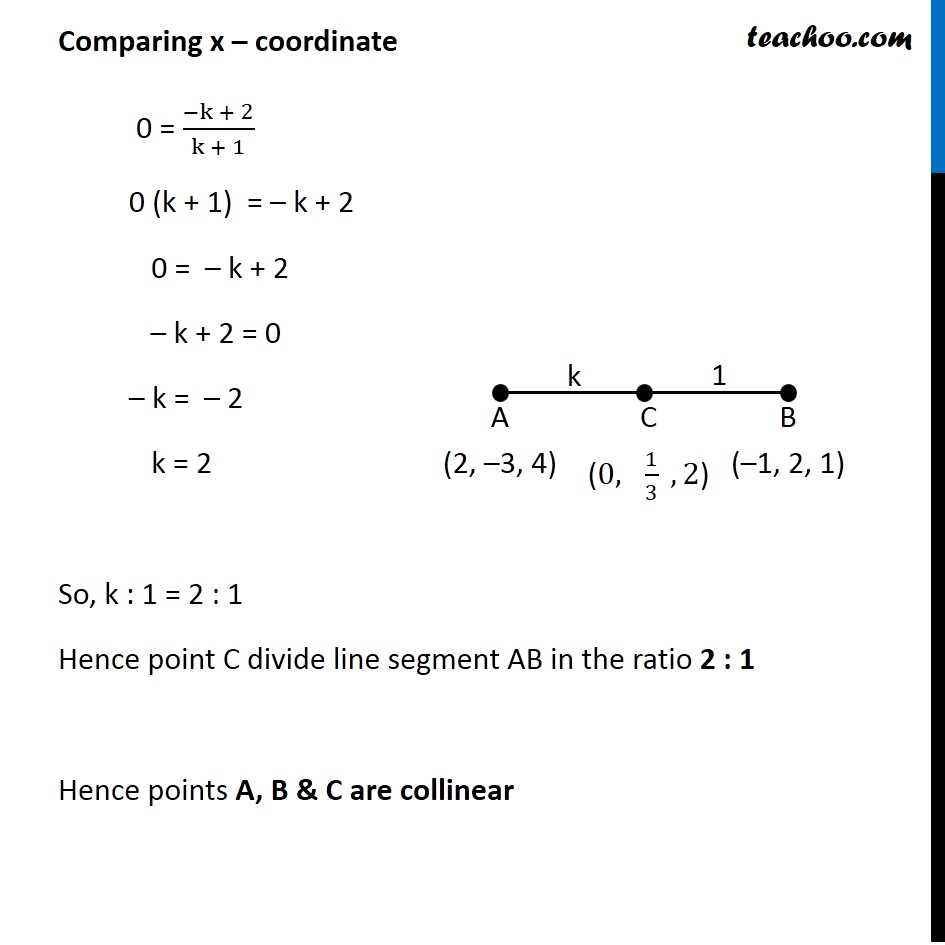Section Formula in 3D Geometry

Chapter 11 Class 11 - Intro to Three Dimensional Geometry
Serial order wiseLearn in your speed, with individual attention - Teachoo Maths 1-on-1 Class

### Transcript

Question 4 Using section formula, show that the points A (2, –3, 4), B (–1, 2, 1) and C (0, 1/3,2) are collinear. Given Points A (2, –3, 4) , B (–1, 2, 1) & C (0, 1/3 ,2) Point A, B & C are collinear if , point C divides AB in some ratio externally or internally. We know that Co-ordinate of point P(x, y ,z) that divides line segment joining (x1, y1, z1) & (x2, y2, z2) in ration m : n is (x, y ,z) = ((mx2 + nx1)/(m + n),(my2 + ny1)/(m + n), (〖𝑚𝑧〗_2 + 〖𝑛𝑧〗_1)/(𝑚 + 𝑛)) Here, let point C (0, 1/3 ,2) divide A(2, –3, 4), B(–1, 2, 1) in the ratio k : 1 Here, m = k , n = 1 x1 = 2, y1 = 3, z1 = 4 x2 = – 1, y2 = 2, z2 = 1 Putting values ("0, " 1/3 ", 2" ) =((k(−1) + 1(2))/(k + 1),(k(2) + 1(3))/(k + 1),(k(1) + 1(4))/(k + 1)) ("0, " 1/3 ", 2" ) =((−k + 2)/(k + 1),(2k + 3)/(k + 1),(k + 4)/(k + 1)) Comparing x – coordinate 0 = (−k + 2)/(k + 1) 0 (k + 1) = – k + 2 0 = – k + 2 – k + 2 = 0 – k = – 2 k = 2 So, k : 1 = 2 : 1 Hence point C divide line segment AB in the ratio 2 : 1 Hence points A, B & C are collinear